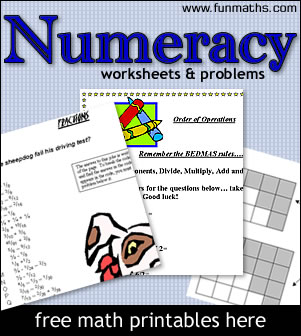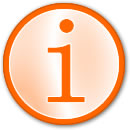# Numeracy Worksheets & Problems

printable worksheets > numeracy worksheets & problems

Page updated : 14 April 2018Below are a number of worksheets covering basic numeracy problems for high school students.

Basic Numeracy Skills include : Arithmetic Skills (Addition, Substraction, Multiplication, Division), Fractions, Decimals, Percentages, Ratio, Calculator Skills, Estimation etc

Arithmetic is the study of numbers, particularly with regard to simple operations like addition, subtraction, division and mulitplication. It is also concerned with using these operations to solve problems.

A fraction is a part of a whole. For example 3/4 or three quarters.

Decimals are a system of representing fractions using a base of ten. For example one half is 1/2 or 5/10 or 0.5.

Percentages show fractions as parts of 100. For exampe 20% is 20 parts per hundred or 20/100.

Ratios are a way of comparing two or more quanties by considering the quotient of the quantities. They are written in the form, for example, as 2:1

High school math students can use these numeracy problems for study purposes. High School Teachers - you're welcome to copy these worksheets for classroom use. Parents - if you'd like to help your child learn math we suggest you start with our math tutorial section before returning to use these worksheets.

Click on any heading to view the worksheet. All worksheets are printable, either as a .gif or .pdf.

## NUMERACY & ARITHMETIC WORKSHEETS

• Using negative numbers between 0 and 100.
• <,> and =
• Solving an expression.
• Year 8 or 9
• Write on? Yes
• Page 1
Page2
Page 3

## Order of Operations.

• A few quick questions.
• Year 8
• Write on? Yes
• Page 1

## ANZAC Maths

• Calculations/percentages
• Year 9 to 11
• Write on? No
• Page 1 (PDF)

## Have you got the power?

• Powers of 2, 3 in a 'numbersearch'.
• Year 9
• Write on? Yes
• Page 1
Page 2

## Order of Operations.

• Calculations using the order of operations.
• Year 7 to 9
• Write on? Yes
• Page 1
Page 2

## Sum to 12.

• General numeracy skills.
• Year 7
• Write on? No
• Page 1

## Long Multiplication

• Multiplying by 10, 100, 1000
• Year 8
• Write on? No
• Page 1 (PDF)

## Directed Numbers

• 60 questions with positive and negative numbers
• Year 8 or 9
• Write on? Yes
• Page 1 (PDF)

• Mortgage and finance research project.
• Use of online information.
• Year 8 to 12
• Write on? No
• Page 1

## Calculator Zapping.

• General numeracy and calculator skills.
• Year 6 or 7
• Write on? No
• Page 1

## Approximations.

• Rounding to one whole unit and one tenth and comparing estimates with actual values
• Year 7 or 8
• Write on? No
• Page 1
Page 2

## Competition - Spend \$1,000,000!!

• Spend one million dollars exactly.
• Year 6 to 8
• Write on? No
• Page 1

## Why did the sheepdog fail his driving test?

• Addition and subtraction of fractions.
• Year 9 or 10
• Write on? Yes
• Page 1

## Toy Soldiers

• Line Ups - linear patterns
• Year 7 or 8
• Write on? Yes
• Page 1
Page 2

## Triangular Numbers.

• A pattern investigation.
• Year 10
• Write on? No
• Page 1

## Investigating Amazing Number Facts.

• Extension activities involving squares, cubes, triangular numbers and more.
• Year 9 to 11
• Write on? No
• Page 1
Page 2
Page 3
Page 4
Page 5

## Percentage Bubbles.

• Calculating the percntage of an amount without a calculator.
• Year 8 or 9
• Write on? Yes
• Page 1

## Fraction of an amount.

• A follow me or loop game calculating halves and quarters.
• Also included ideas for an algebra and fraction / decimal game.
• Year 8 or 9
• Write on? No
• Page 1
Page 2

## Using a calculator.

• Use a calculator to make invented words.
• Year 8 or 9
• Write on? Yes
• Page 1
Page 2

## Manic Mazes.

• Use calculators and explore using 4 rules with whole and decimal number
• Year 7
• Write on? Yes
• Page 1

## Fraction and ratio worksheet.

• Reduce to simplest form.
• Sharing by ratio.
• Equivalent fractions and ratios.
• Ratios in real life
• Year 9 to 11
• Write on? Yes
• Page 1

## Equivalent Fractions.

• Introduction to equivalent fractions.
• Year 8 or 9
• Write on? Yes
• Page 1

## Up-side-down Crossword.

• Calculator skills.
• Year 9 or 10
• Write on? Yes
• Page 1
Page 2

## Squares.

• An integer game for 2 to 4 players.
• Year 9 to 11
• Write on? Yes
• Page 1
Page 2

## Earth Facts.

• Scientific notation.
• Year 10 or 11
• Write on? Yes
• Page 1

## At Work.

• Fraction word problems.
• Year 10 or 11
• Write on? No
• Page 1

## The Binary System of Counting.

• Converting decimal numbers to binary numbers with notes and examples.
• Year 10 or 11
• Write on? Yes
• Page 1

## Percentage Puzzle.

• Calculating percentages.
• Percentage increase and decrease.
• Year 11
• Write on? Yes
• Page 1
Page 2

## Greatest Common Factor.

• Determining the GCF.
• Prime Factorisation.
• Year 8 or 9
• Write on? Yes
• Page 1

## Directed Numbers.

• 60 quick questions on intergers.
• Year 8 or 9
• Write on? Yes
• Page 1

## Long Multiplication.

• Complete Multiplication
• Long Multiplication
• Year 8 or 9
• Write on? Yes
• Page 1

## Anzac Maths.

• Calculations / Percentages
• New Zealand Specific
• Year 9 to 11
• Write on? No
• Page 1

## Negative Number Game.

• Multiplying directed numbers.
• A game for 2 players.
• Year 8 to 10
• Write on? Yes
• Page 1

• Year 9
• Write on? Yes
• Page 1
Page 2

## Number Operations.

• Grouped all Number Operations questions from Year 1996 to 2000 of Sequential I Regents.
• Notes and questions covering order of operations, exponents, scientific notation, rational and irrational numbers and more.
• Year 10 or 11
• Write on? Yes
• Page 1
Page 2
Page 3
Page 4
Page 5

## ARITHMETIC & NUMERACY TUTORIALS

• Order of Operations
In a math calculation the order you do the operations (add, subtract, multiply, divide and brackets) is important.
This tutorial is suitable for students in Year 7 to 9.
• Directed Numbers
Directed numbers are signed numbers i.e. numbers with a positive or plus sign (+) in front of them or a negative or minus sign (-) in front of them  e.g.  +6, -2, +7 etc.  All these whole numbers (and 0) grouped together are called integers.
How do you add, subtract, multiply and divide directed numbers? View the tutorial.
This tutorial is suitable for students in Year 9 or 10.

## FRACTIONS TUTORIALS

• An Introduction to Fractions
What are fractions? How do you express a diagram as a fraction? What are the parts of a fraction called?
This tutorial is suitable for students in Year 8 or 9.
• Equivalent Fractions
What are equivalent fractions, improper fractions and mixed numbers? How to simplify a fraction.
This tutorial is suitable for students in Year 9.
• Fractions, Decimals & Percentages
How to convert fractions to decimals and percentages.
This tutorial is suitable for students in Year 9.
• Ratios
What are ratios and how to simplify them.
This tutorial is suitable for students in Year 9.

##A note about year levels

Where appropriate each worksheet is given a year level that it is applicable to. As we're all in different countries the year level corresponds to the number of years at school. So, for example, a worksheet for Year 11 is for students in their 11th year of school.
Worksheets for earlier or later years may still be suitable for you.

Please note : This is a free service and these worksheets are supplied on 'as is' basis. We will not enter into any correspondence on the content of the worksheets, errors, answers or tuition.